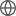Old Web
English
# First HETDEX Spectroscopic Determinations of Ly$\alpha$ and UV Luminosity Functions at $z=2-3$: Bridging a Gap Between Faint AGN and Bright Galaxies.
We present Ly$\alpha$ and ultraviolet-continuum (UV) luminosity functions (LFs) of galaxies and active galactic nuclei (AGN) at $z=2.0-3.5$ determined by the un-targetted optical spectroscopic survey of the Hobby-Eberly Telescope Dark Energy Experiment (HETDEX). We combine deep Subaru imaging with HETDEX spectra resulting in $11.4$ deg$^2$ of fiber-spectra sky coverage, obtaining $18320$ galaxies spectroscopically identified with Ly$\alpha$ emission, $2126$ of which host type 1 AGN showing broad (FWHM$~>1000$ km s$^{-1}$) Ly$\alpha$ emission lines. We derive the Ly$\alpha$ (UV) LF over 2 orders of magnitude covering bright galaxies and AGN in $\log L_\mathrm{Ly\alpha}/\mathrm{[erg~s^{-1}]}=43.3-45.2$ ($-27 -21$) type 1 AGN increases from $z\sim2$ to $z\sim0$ as opposed to the evolution of bright ($M_\mathrm{UV}<-21$) type 1 AGN, suggesting the AGN downsizing in the rest-frame UV luminosity.